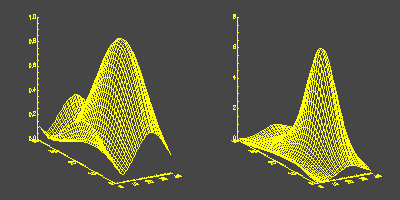# Exaggerate the Z-Axis

To exaggerate the Z-axis of a surface plot try multiplying your data by an exponential factor. Here is an example program that indicates what I mean.

```   PRO EXAMPLE

; Create some data.

peak = SHIFT(DIST(40),40/2+5, 40/2-5)
peak = EXP(-(peak/15)^2)

; Open a window.

WINDOW, /FREE, XSIZE=600, YSIZE=300

; Side-by-side plots.

!P.MULTI = [0,2,1]

; Display the data.

SURFACE, peak
SURFACE, peak * EXP(peak * 2.0)

; Regular plotting.

!P.MULTI = 0
END
```

Your output should look like the display below.[Return to IDL Programming Tips]